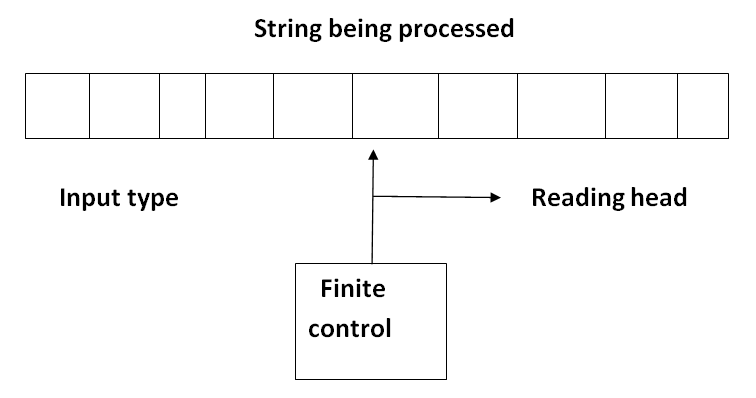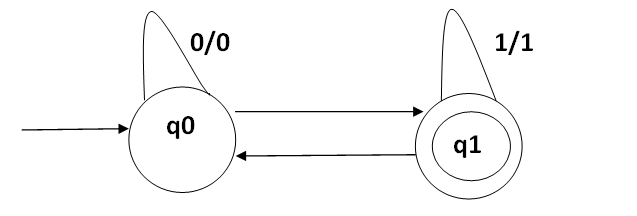# Finite-state Machine: What It Is, Components, and Types

In this tutorial, we will learn about the finite-state machine, its components, and types in Discrete Mathematics. By Prerana Jain Last updated : May 09, 2023

## What is Finite-state Machine in Discrete Mathematics?

A finite-state machine (FSM) is similar to a finite-state automation (FSA) except that the finite state machine "prints" an output using an output alphabet distinct from the input alphabet. The final definition is as follow:

A finite state machine (also called complete sequential machine ) M( A, S, Z, S0, f, g) consists of six points.

1. A finite set A of input alphabet.
2. A finite set S of internal state.
3. A finite set z of output symbol.
4. An initial state S0 in S.
5. A next-state function f from S X A into S.
6. An output function g from S X A into Z.

## Components of Finite-state Machine

### 1. Input Type

The input type is divided into a square and each square contains a single symbol from the input alphabet X. The end squares of the tape contain end markers at the left end and \$ at the right end. The absence of end markers in the input tape indicates that there is an infinite length of the tape. The left to right sequence of symbol between the end markers is the input string to be processed.

The head examines only one square at a time and can move on square either to the left or to the right. For further analysis are restrict the movement of R head only to the right side.

### 3. Finite Control

The input to the finite control will be usually symboled under the R-head say a or the present state of the machine say q to give the following output:

• A motion of R head along the tape to the next square.
• The next state of the finite state machine given by & (q, a).

### 4. Transition System

The finite labeled graph in which a state is represented by the vertex or node and the directed edges indicates the transition of a state and the edges are labeled with input/output is known as transition graph or a transition system.

In the normal transition diagram, the initial state is represented by a circle with an arrow pointing towards it, the final state by two concentric circles and the other states are represented by just a circle.## Types of Finite Automata (Finite-state Machine)

There are two types of finite automata (finite-state machine):

### 1. Deterministic Finite Automata

Some moves of the machine can be uniquely determined by the input symbol and present state. The DFA can be defined with 5 tuples (Q, X, &, q0, F). When,

• Q - is a finite non-empty set of states.
• X - is a finite non-empty set of input called input alphabet.
• & - is a function which maps Q x X into q and is usually called direct transition function. This is the function which describes the change of states driving the transition. This mapping is usually represented by a transition table or a transition diagram.
• Qo E Q - is the initial state and,
• F C Q - is the set of final states. It is assumed here that there may be more than one final state.

### 2. Non-deterministic Finite Automata

Some moves of the machine can not uniquely determine by the input symbol and present state. The NDFA can be defined with 5 tuples. (Q, X, f, q0, F). Where,

• Q - is a finite non-empty set of states.
• X - is a finite non-empty set of inputs.
• & - is the transition function mapping from Q x X into 2Q which is the power set of Q, the set of all subset of Q.
• q0 E Q - is the initial state.
• F C Q - is the set of final states.

## Difference Between the Deterministic and Non-deterministic Automata

The difference between the deterministic and non- deterministic automata is only in &. For deterministic automation, the outcome is a state i.e. an element of Q for non- deterministic automation the outcome is a subset of Q.